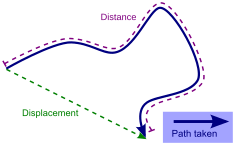# Displacement vector facts for kids

Kids Encyclopedia FactsThe displacement vector (in green) measures the distance from some reference point. In most cases, this is smaller than actual distance travelled. Simply speaking, the green arrow is shorter than the blue one.

A Displacement vector is a concept from mathematics. It is a vector. It shows the direction and distance travelled. It is often used in physics to display the speed, acceleration and distance an object travelled (compared to a reference point or an object's initial position).Displacement vector Facts for Kids. Kiddle Encyclopedia.